# Experimenting with Drawing Combinators

I’m working on a functional TypeScript library for drawing to a canvas inspired by parser combinators. Let’s try it out by making some generative art! If you haven’t heard of creative coding, this talk by Tim Holman is a great intro.

First we need to setup a project. We will make an `index.html` with a canvas, a `main.ts` that renders our sketch to the canvas, and a `sketch.ts` that defines our sketch.

``````// main.ts

import { render } from './drawing-combinators';
import sketch from './sketch';

const canvas = document.getElementById('canvas') as HTMLCanvasElement;
render(canvas, sketch);``````
``````// sketch.ts

import * as D from './drawing-combinators';

export default [D.fillColor('white', D.fillRect(0, 0, 100, 100)), D.strokeCircle(50, 50, 50)];``````

The `render` function expects a 100x100 by sketch. It then scales to fit the canvas and renders the sketch.

The above code results in a circle on a white background.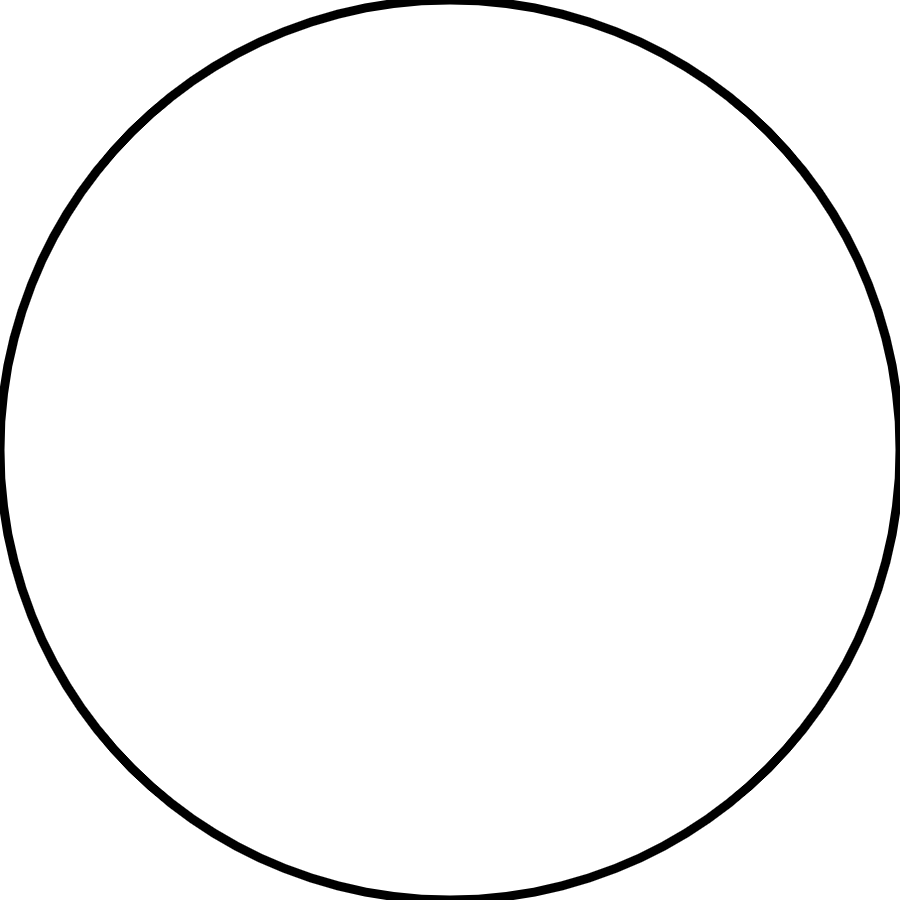Now we can edit `sketch.ts` to draw whatever we want. Let’s start with a classic, 10 PRINT.

``````import * as D from './drawing-combinators';

const range = (amount: number) => [...Array(amount).keys()];

const line = (size: number) =>
Math.random() < 0.5 ? D.strokeLine(0, 0, size, size) : D.strokeLine(0, size, size, 0);

const grid = (size: number) =>
range(size).map((x) =>
range(size).map((y) => D.translate((x * 100) / size, (y * 100) / size, line(100 / size)))
);

export default [D.fillColor('white', D.fillRect(0, 0, 100, 100)), grid(20)];``````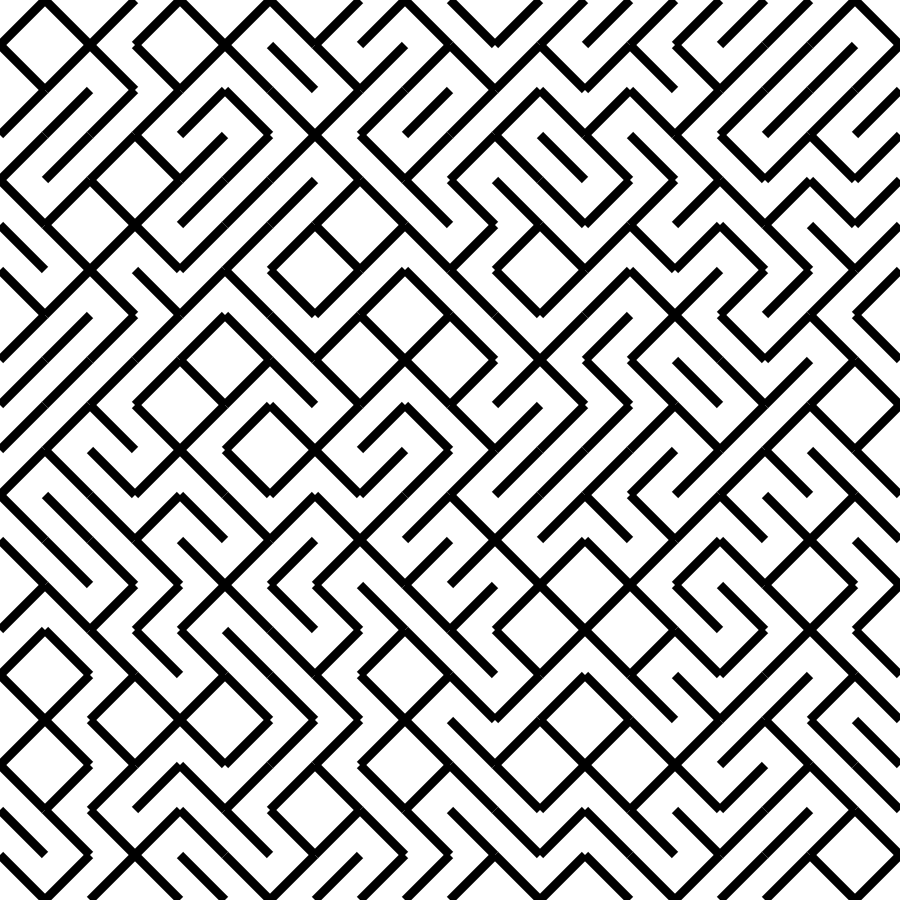The code isn’t as concise as it is on the Commodore 64, but this will allow for some fun additions. Let’s make the lines horizontal and vertical.

``````const line = (size: number) =>
Math.random() < 0.5
? D.strokeLine(0, size / 2, size, size / 2)
: D.strokeLine(size / 2, 0, size / 2, size);``````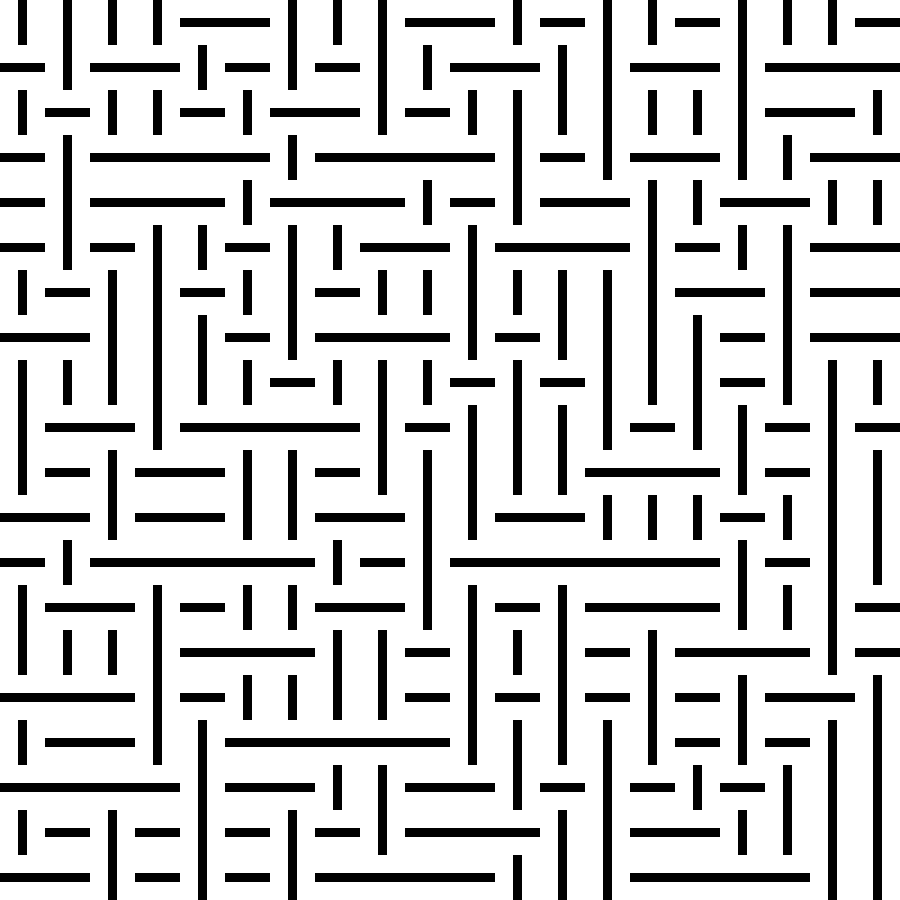What if all 4 types of lines are possible?

``````const randomChoice = <T>(choices: T[]) => choices[Math.floor(Math.random() * choices.length)];

const line = (size: number) =>
randomChoice([
D.strokeLine(0, 0, size, size),
D.strokeLine(0, size, size, 0),
D.strokeLine(0, size / 2, size, size / 2),
D.strokeLine(size / 2, 0, size / 2, size)
]);``````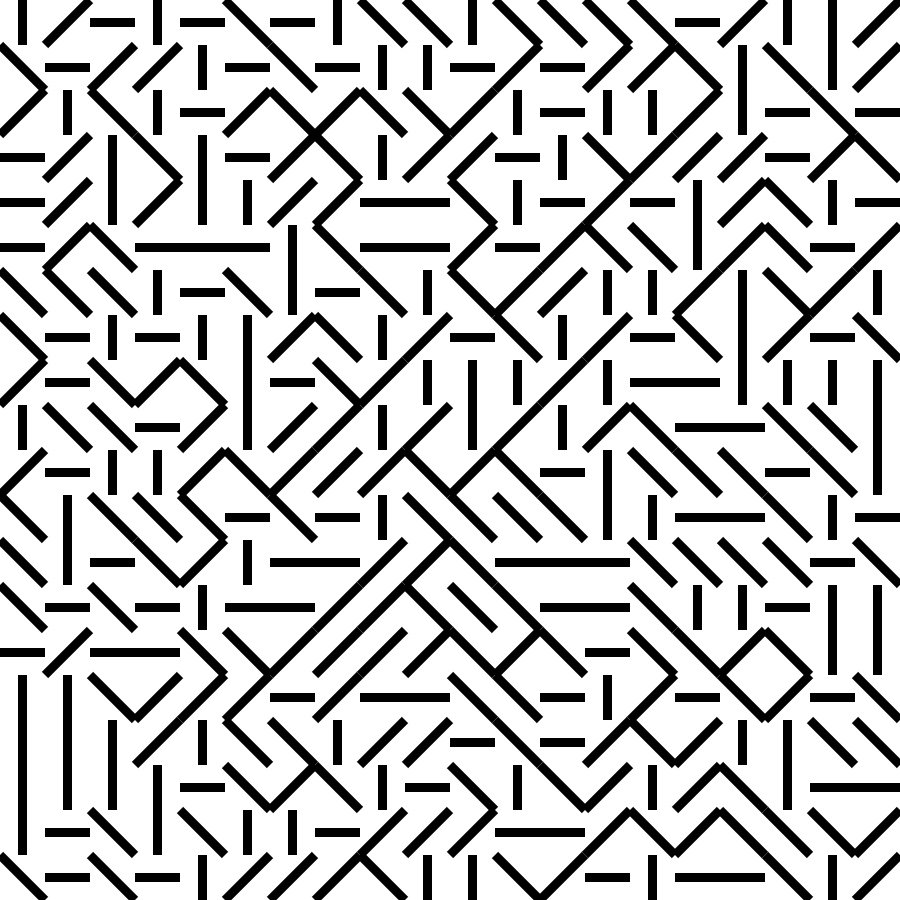What if we clip it to a circle?

``````export default [
D.fillColor('white', D.fillRect(0, 0, 100, 100)),
D.clipCircle(50, 50, 40, grid(20))
];``````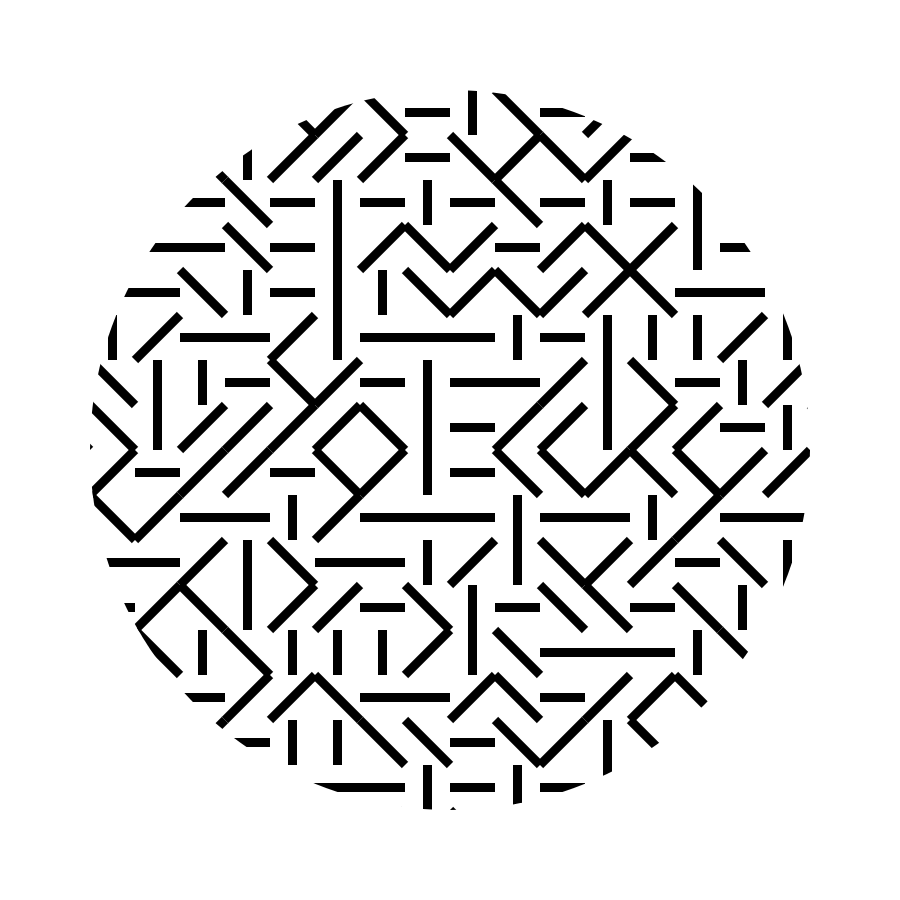That’s pretty cool! Now let’s change it up entirely and try something recursive.

``````import * as D from './drawing-combinators';

const centeredSquare = (x: number, y: number, size: number) =>
D.translate(x - size / 2, y - size / 2, D.strokeRect(0, 0, size, size));

const DIST_MULTIPLIER = 0.027;
const ANGLE_CHANGE_SPEED = 0.4;
const SIZE_CHANGE_SPEED = 0.8;

const spiral = (x: number, y: number, size: number, angle: number) =>
size < 40
? [
D.translate(x, y, D.rotateAround(angle, size / 2, size / 2, centeredSquare(0, 0, size))),
...spiral(
x + Math.cos(angle) * DIST_MULTIPLIER * size ** 2,
y + Math.sin(angle) * DIST_MULTIPLIER * size ** 2,
size + SIZE_CHANGE_SPEED,
angle + ANGLE_CHANGE_SPEED
)
]
: [];

export default [
D.fillColor('white', D.fillRect(0, 0, 100, 100)),
D.strokeWidth(1 / 3, spiral(50, 50, 1, 0))
];``````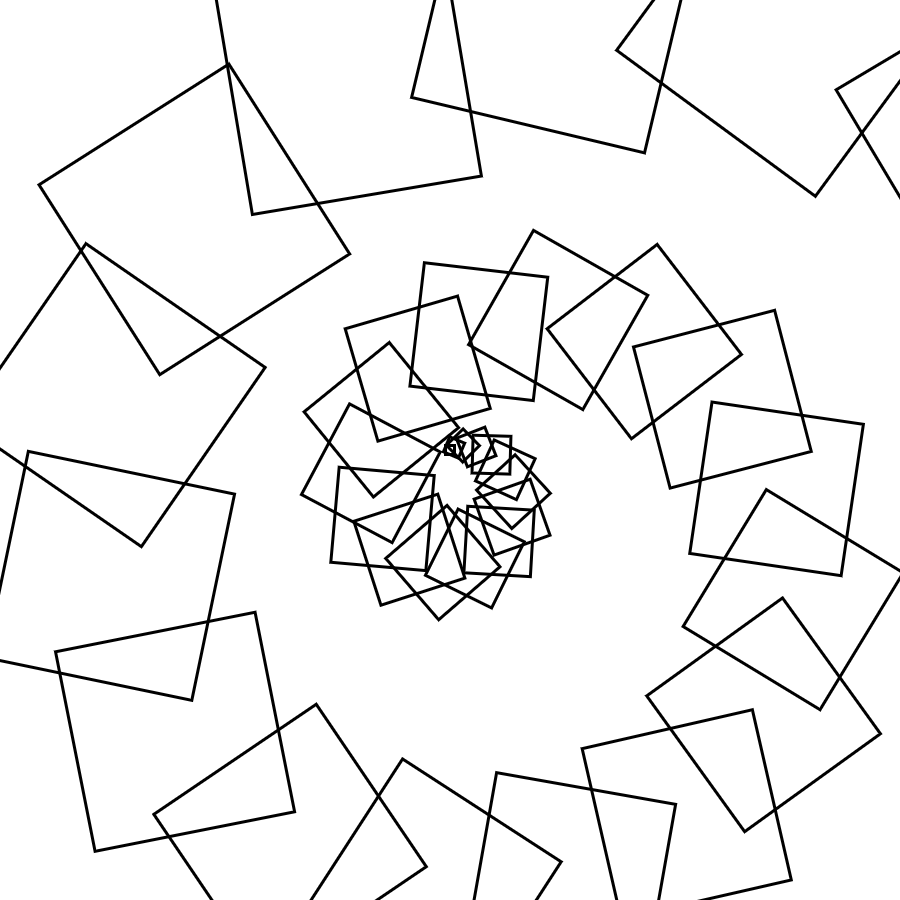Nice! I think this was a successful experiment. Creative coding is always fun, but this library made it more fun. Changing requirements on the fly is common when creative coding, so functional programming is a great fit. Our code becomes composable and reusable by default.

Website made with ❤️ by me.